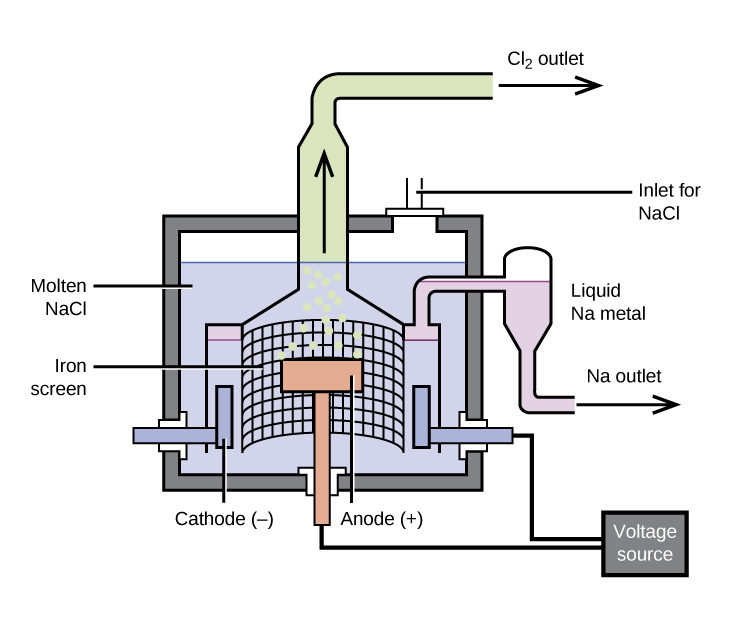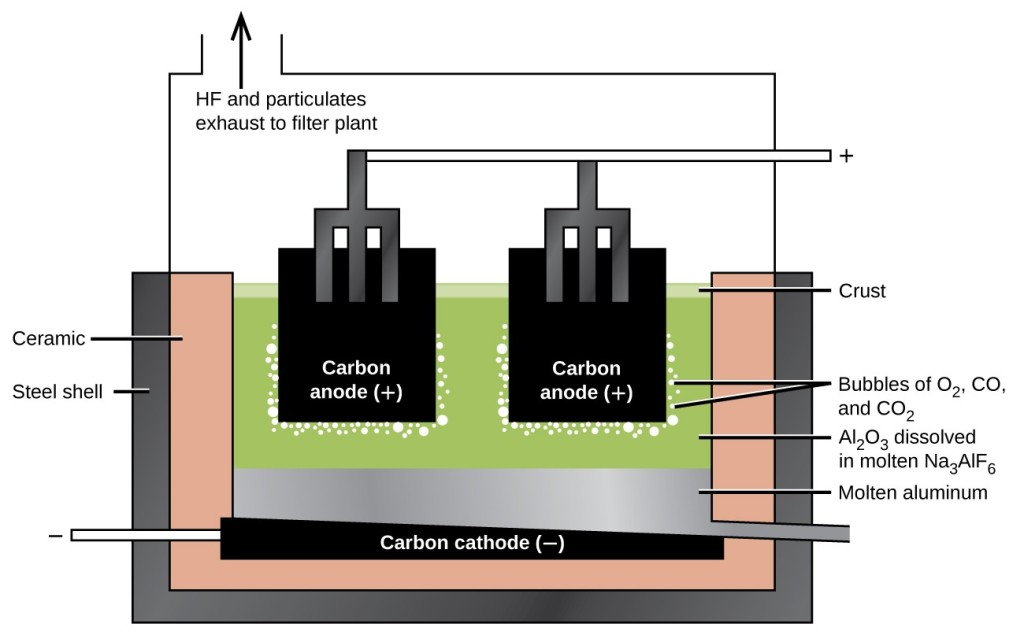## Occurrence and Preparation of the Representative Metals

### Learning Outcomes

• Identify natural sources of representative metals
• Describe electrolytic and chemical reduction processes used to prepare these elements from natural sources

Because of their reactivity, we do not find most representative metals as free elements in nature. However, compounds that contain ions of most representative metals are abundant. In this section, we will consider the two common techniques used to isolate the metals from these compounds—electrolysis and chemical reduction.

These metals primarily occur in minerals, with lithium found in silicate or phosphate minerals, and sodium and potassium found in salt deposits from evaporation of ancient seas and in silicates. The alkaline earth metals occur as silicates and, with the exception of beryllium, as carbonates and sulfates. Beryllium occurs as the mineral beryl, Be3Al2Si6O18, which, with certain impurities, may be either the gemstone emerald or aquamarine. Magnesium is in seawater and, along with the heavier alkaline earth metals, occurs as silicates, carbonates, and sulfates. Aluminum occurs abundantly in many types of clay and in bauxite, an impure aluminum oxide hydroxide. The principle tin ore is the oxide cassiterite, SnO2, and the principle lead and thallium ores are the sulfides or the products of weathering of the sulfides. The remaining representative metals occur as impurities in zinc or aluminum ores.

## Electrolysis

Ions of metals in of groups 1 and 2, along with aluminum, are very difficult to reduce; therefore, it is necessary to prepare these elements by electrolysis, an important process discussed in the chapter on electrochemistry. Briefly, electrolysis involves using electrical energy to drive unfavorable chemical reactions to completion; it is useful in the isolation of reactive metals in their pure forms. Sodium, aluminum, and magnesium are typical examples.

### The Preparation of Sodium

The most important method for the production of sodium is the electrolysis of molten sodium chloride; the set-up is a Downs cell, shown in Figure 1. The reaction involved in this process is:

$\text{2NaCl}\left(l\right)\,\,\,{\underset{600^{\circ}\text{C}}{\xrightarrow{\text{electrolysis}}}}\,\,\,\text{2Na}\left(l\right)+{\text{Cl}}_{2}\left(g\right)$

The electrolysis cell contains molten sodium chloride (melting point 801 °C), to which calcium chloride has been added to lower the melting point to 600 °C (a colligative effect). The passage of a direct current through the cell causes the sodium ions to migrate to the negatively charged cathode and pick up electrons, reducing the ions to sodium metal. Chloride ions migrate to the positively charged anode, lose electrons, and undergo oxidation to chlorine gas. The overall cell reaction comes from adding the following reactions:

$\begin{array}{rrcl}\text{at the cathode:}&{\text{2Na}}^{+}+{\text{2e}}^{-}&\longrightarrow&\text{2Na}\left(l\right)\\ \text{at the anode:}&{\text{2Cl}}^{-}&\longrightarrow&{\text{Cl}}_{2}\left(g\right)+{\text{2e}}^{-}\\ \\ \text{overall change:}&{\text{2Na}}^{+}+{\text{2Cl}}^{-}&\longrightarrow&\text{2Na}\left(l\right)+{\text{Cl}}_{2}\left(g\right)\end{array}$

Separation of the molten sodium and chlorine prevents recombination. The liquid sodium, which is less dense than molten sodium chloride, floats to the surface and flows into a collector. The gaseous chlorine goes to storage tanks. Chlorine is also a valuable product.Figure 1. Pure sodium metal is isolated by electrolysis of molten sodium chloride using a Downs cell. It is not possible to isolate sodium by electrolysis of aqueous solutions of sodium salts because hydrogen ions are more easily reduced than are sodium ions; as a result, hydrogen gas forms at the cathode instead of the desired sodium metal. The high temperature required to melt NaCl means that liquid sodium metal forms.

### The Preparation of Aluminum

The preparation of aluminum utilizes a process invented in 1886 by Charles M. Hall, who began to work on the problem while a student at Oberlin College in Ohio. Paul L. T. Héroult discovered the process independently a month or two later in France. In honor to the two inventors, this electrolysis cell is known as the Hall–Héroult cell. The Hall–Héroult cell is an electrolysis cell for the production of aluminum. Figure 2 illustrates the Hall–Héroult cell.

The production of aluminum begins with the purification of bauxite, the most common source of aluminum. The reaction of bauxite, AlO(OH), with hot sodium hydroxide forms soluble sodium aluminate, while clay and other impurities remain undissolved:

$\text{AlO}\left(\text{OH}\right)\left(s\right)+\text{NaOH}\left(aq\right)+{\text{H}}_{2}\text{O}\left(l\right)\longrightarrow\text{Na}\left[\text{Al}{\left(\text{OH}\right)}_{4}\right]\left(aq\right)$

After the removal of the impurities by filtration, the addition of acid to the aluminate leads to the reprecipitation of aluminum hydroxide:

$\text{Na}\left[\text{Al}{\left(\text{OH}\right)}_{4}\right]\left(aq\right)+{\text{H}}_{3}{\text{O}}^{+}\left(aq\right)\longrightarrow\text{Al}{\left(\text{OH}\right)}_{3}\left(s\right)+{\text{Na}}^{+}\left(aq\right)+{\text{2H}}_{2}\text{O}\left(l\right)$

The next step is to remove the precipitated aluminum hydroxide by filtration. Heating the hydroxide produces aluminum oxide, Al2O3, which dissolves in a molten mixture of cryolite, Na3AlF6, and calcium fluoride, CaF2. Electrolysis of this solution takes place in a cell like that shown in Figure 2. Reduction of aluminum ions to the metal occurs at the cathode, while oxygen, carbon monoxide, and carbon dioxide form at the anode.Figure 2. An electrolytic cell is used for the production of aluminum. The electrolysis of a solution of cryolite and calcium fluoride results in aluminum metal at the cathode, and oxygen, carbon monoxide, and carbon dioxide at the anode.

### The Preparation of Magnesium

Magnesium is the other metal that is isolated in large quantities by electrolysis. Seawater, which contains approximately 0.5% magnesium chloride, serves as the major source of magnesium. Addition of calcium hydroxide to seawater precipitates magnesium hydroxide. The addition of hydrochloric acid to magnesium hydroxide, followed by evaporation of the resultant aqueous solution, leaves pure magnesium chloride. The electrolysis of molten magnesium chloride forms liquid magnesium and chlorine gas:

$\begin{array}{rcl}{\text{MgCl}}_{2}\left(aq\right)+\text{Ca}{\left(\text{OH}\right)}_{2}\left(aq\right)&\longrightarrow&\text{Mg}{\left(\text{OH}\right)}_{2}\left(s\right)+{\text{CaCl}}_{2}\left(aq\right)\\\text{Mg}{\left(\text{OH}\right)}_{2}\left(s\right)+\text{2HCl}\left(aq\right)&\longrightarrow&{\text{MgCl}}_{2}\left(aq\right)+{\text{2H}}_{2}\text{O}\left(l\right)\\{\text{MgCl}}_{2}\left(l\right)&\longrightarrow&\text{Mg}\left(l\right)+{\text{Cl}}_{2}\left(g\right)\end{array}$

Some production facilities have moved away from electrolysis completely. In the next section, we will see how the Pidgeon process leads to the chemical reduction of magnesium.

## Chemical Reduction

It is possible to isolate many of the representative metals by chemical reduction using other elements as reducing agents. In general, chemical reduction is much less expensive than electrolysis, and for this reason, chemical reduction is the method of choice for the isolation of these elements. For example, it is possible to produce potassium, rubidium, and cesium by chemical reduction, as it is possible to reduce the molten chlorides of these metals with sodium metal. This may be surprising given that these metals are more reactive than sodium; however, the metals formed are more volatile than sodium and can be distilled for collection. The removal of the metal vapor leads to a shift in the equilibrium to produce more metal (see how reactions can be driven in the discussions of Le Châtelier’s principle in the chapter on fundamental equilibrium concepts).

The production of magnesium, zinc, and tin provide additional examples of chemical reduction.

### The Preparation of Magnesium

The Pidgeon process involves the reaction of magnesium oxide with elemental silicon at high temperatures to form pure magnesium:

$\text{Si}\left(s\right)+\text{2MgO}\left(s\right)\stackrel{\Delta}{\longrightarrow }{\text{SiO}}_{2}\left(s\right)+\text{2Mg}\left(g\right)$

Although this reaction is unfavorable in terms of thermodynamics, the removal of the magnesium vapor produced takes advantage of Le Châtelier’s principle to continue the forward progress of the reaction. Over 75% of the world’s production of magnesium, primarily in China, comes from this process.

### The Preparation of Zinc

Zinc ores usually contain zinc sulfide, zinc oxide, or zinc carbonate. After separation of these compounds from the ores, heating in air converts the ore to zinc oxide by one of the following reactions:

$\begin{array}{rll}\text{2ZnS}\left(s\right)+{\text{3O}}_{2}\left(g\right)&\stackrel{\Delta}{\longrightarrow }&\text{2ZnO}\left(s\right)+{\text{2SO}}_{2}\left(g\right)\\{\text{ZnCO}}_{3}\left(s\right)&\stackrel{\Delta}{\longrightarrow }&\text{ZnO}\left(s\right)+{\text{CO}}_{2}\left(g\right)\end{array}$

Carbon, in the form of coal, reduces the zinc oxide to form zinc vapor:

$\text{ZnO}\left(s\right)+\text{C}\left(s\right)\rightarrow\text{Zn}\left(g\right)+\text{CO}\left(g\right)$

The zinc can be distilled (boiling point 907 °C) and condensed. This zinc contains impurities of cadmium (767 °C), iron (2862 °C), lead (1750 °C), and arsenic (613 °C). Careful redistillation produces pure zinc. Arsenic and cadmium are distilled from the zinc because they have lower boiling points. At higher temperatures, the zinc is distilled from the other impurities, mainly lead and iron.

### The Preparation of Tin

The ready reduction of tin(IV) oxide by the hot coals of a campfire accounts for the knowledge of tin in the ancient world. In the modern process, the roasting of tin ores containing SnO2 removes contaminants such as arsenic and sulfur as volatile oxides. Treatment of the remaining material with hydrochloric acid removes the oxides of other metals. Heating the purified ore with carbon at temperature above 1000 °C produces tin:

${\text{SnO}}_{2}\left(s\right)+\text{2C}\left(s\right)\stackrel{\Delta}{\longrightarrow }\text{Sn}\left(s\right)+\text{2CO}\left(g\right)$

The molten tin collects at the bottom of the furnace and is drawn off and cast into blocks.

### Key Concepts and Summary

Because of their chemical reactivity, it is necessary to produce the representative metals in their pure forms by reduction from naturally occurring compounds. Electrolysis is important in the production of sodium, potassium, and aluminum. Chemical reduction is the primary method for the isolation of magnesium, zinc, and tin. Similar procedures are important for the other representative metals.

### Try It

1. Write an equation for the reduction of cesium chloride by elemental calcium at high temperature.
2. Why is it necessary to keep the chlorine and sodium, resulting from the electrolysis of sodium chloride, separate during the production of sodium metal?
3. Give balanced equations for the overall reaction in the electrolysis of molten lithium chloride and for the reactions occurring at the electrodes. You may wish to review the chapter on electrochemistry for relevant examples.
4. The electrolysis of molten sodium chloride or of aqueous sodium chloride produces chlorine.
Calculate the mass of chlorine produced from 3.00 kg sodium chloride in each case. You may wish to review the chapter on electrochemistry for relevant examples.
5. What mass, in grams, of hydrogen gas forms during the complete reaction of 10.01 g of calcium with water?
6. How many grams of oxygen gas are necessary to react completely with 3.01 × 1021 atoms of magnesium to yield magnesium oxide?
7. Magnesium is an active metal; it burns in the form of powder, ribbons, and filaments to provide flashes of brilliant light. Why is it possible to use magnesium in construction?
8. Why is it possible for an active metal like aluminum to be useful as a structural metal?
9. Describe the production of metallic aluminum by electrolytic reduction.
10. What is the common ore of tin and how is tin separated from it?
11. A chemist dissolves a 1.497-g sample of a type of metal (an alloy of Sn, Pb, Sb, and Cu) in nitric acid, and metastannic acid, H2SnO3, is precipitated. She heats the precipitate to drive off the water, which leaves 0.4909 g of tin(IV) oxide. What was the percentage of tin in the original sample?
12. Consider the production of 100 kg of sodium metal using a current of 50,000 A, assuming a 100% yield.
1. How long will it take to produce the 100 kg of sodium metal?
2. What volume of chlorine at 25 °C and 1.00 atm forms?
13. What mass of magnesium forms when 100,000 A is passed through a MgCl2 melt for 1.00 h if the yield of magnesium is 85% of the theoretical yield?

## Glossary

chemical reduction: method of preparing a representative metal using a reducing agent

Downs cell: electrochemical cell used for the commercial preparation of metallic sodium (and chlorine) from molten sodium chloride

Hall–Héroult cell: electrolysis apparatus used to isolate pure aluminum metal from a solution of alumina in molten cryolite

Pidgeon process: chemical reduction process used to produce magnesium through the thermal reaction of magnesium oxide with silicon